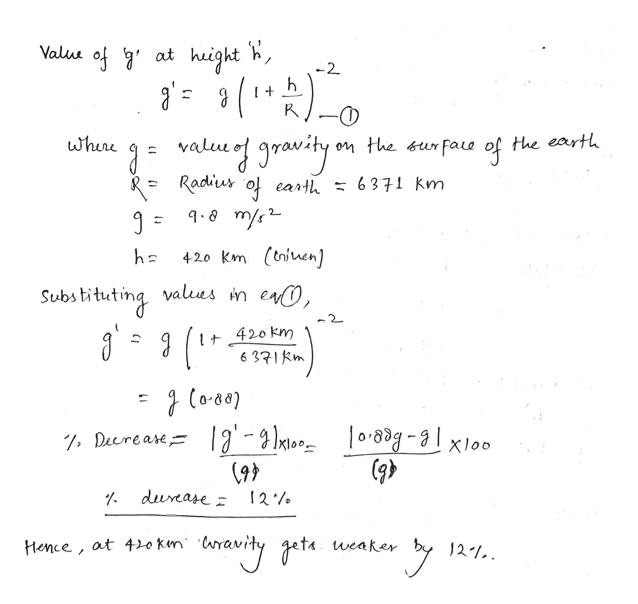# Convince them that it isn't so by calculating how much weaker (in %) gravity is 420 km above the Earth's surface. Express your answer using three significant figures.The gravity is weaker by?

Question
163 views

Convince them that it isn't so by calculating how much weaker (in %) gravity is 420 km above the Earth's surface.

The gravity is weaker by?

check_circle

Step 1

Given:

Height, h = 420 km

Step 2

Calculating value of percentage decrease in the value of gravity at a heigh...help_outlineImage TranscriptioncloseValu of ' at height -2 whure value on the &urFaue Hhe earth of Radius o eart = q-8 m/s 6371 km 420 km (tniuen] bstituting valus n en, 2 420 km 6 371 km lt 0.8d-loo . Deereare a' - 2 Ikoo (93 durcase : Hence, at 420 Ken 'ara weaker 21. fullscreen

### Want to see the full answer?

See Solution

#### Want to see this answer and more?

Solutions are written by subject experts who are available 24/7. Questions are typically answered within 1 hour.*

See Solution
*Response times may vary by subject and question.
Tagged in

### Physics